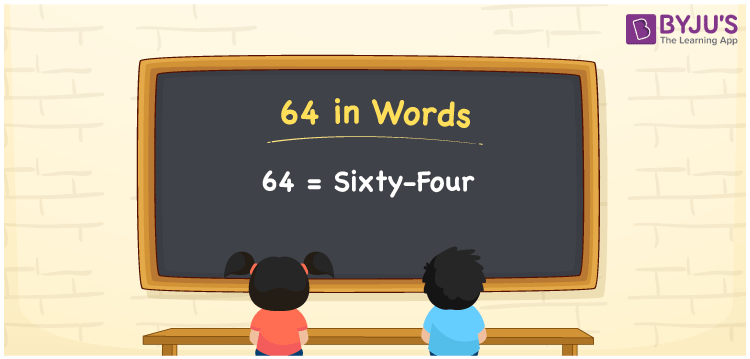# 64 in Words

64 in words can be written as Sixty-four. You will get a clear idea of how numbers can be written using words. For example, if you buy 1 kg of sugar for Rs. 64 at the grocery store, then you can say that “I bought 1 kg of sugar for Sixty-four Rupees at the grocery store”. The numbers in words are basically written using the English alphabet. So, 64 can be read as “Sixty-four” in English.

 64 in words Sixty-four Sixty-four in Numbers 64

## 64 in English Words## How to Write 64 in Words?

This section will provide complete details of the place value chart for 64 in an understandable format. The place value of the two digits is indicated below in the table.

 Tens Ones 6 4

The expanded form of 64 is given here:

6 × Ten + 4 × One

= 6 × 10 + 4 × 1

= 60 + 4

= 64

= Sixty-four

Hence, 64 in words is written as Sixty-four.

64 is a natural number that precedes 65 and succeeds 63.

64 in words – Sixty-four

Is 64 an odd number? – No

Is 64 an even number? – Yes

Is 64 a perfect square number? – Yes

Is 64 a perfect cube number? – Yes

Is 64 a prime number? – No

Is 64 a composite number? – Yes

## Frequently Asked Questions on 64 in Words

Q1

### How should you write 64 in words?

64 can be written as “Sixty-four” in words.
Q2

### What is the value of 70 minus 6?

The value of 70 minus 6 is 64. Therefore, 64 in words is Sixty-four.
Q3

### Is 64 an even number?

Yes, 64 is an even number as it is completely divisible by 2.